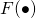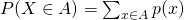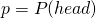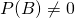# Discrete Random VariablesAs usual, definitions first.

The cumulative distribution function (CDF),of a random variable, X, is defined byA discrete random variable, X, has probability mass function (PMF),, ifand for all events A we haveThe expected value of a discrete random variable, X is given byThe variance of an random variable, X, is defined asNow we can look at some examples of discrete random variables.

Binomial Distribution

We say the X has a binomial distribution, that is,, ifFor example, X can represent the number of heads in n independent coin tosses, where. We have that the meanand varianceA simpler case of binomial where there is only one event is called the Bernoulli distribution.

I’ll give a simple illustration of binomial model used in finance. This was also used in finance engineering in the beginning.
Suppose a fund manager outperforms the market in a given year with probability p and under performs the market with probability 1 – p. She has a track record of 10 years and has outperformed the market in 8 out of 10 years. We also note that performance in any one year is independent of performance in other years.
From this illustration, we note that there are only two outcomes, she outperforms or underperforms. We can let X be the number of outperforming years. Assuming the fund manager has no skill,and we can findto find out the probability that she outperforms at least 8 out of 10 years.
An extension here will be to consider there are M fund managers instead of 1 now.

Poisson Distribution

We say that X has adistribution ifNext, we look at Bayes Theorem, also known as “conditional probability” in H2 Mathematics.

Let A and B be two events for which. Thenwhere‘s form a partition of the sample space.

Not readable? Change text.